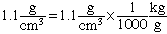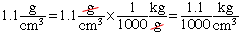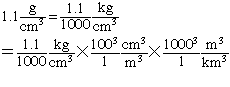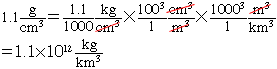Name: Matt Question: I am having trouble finding a workable equation for the following problem, particularly the conversion of two systems of measurement. The question states that there are 27 grams of salt in a kilogram of seawater. And it asks how many tonnes (1 tonne = 1000 kilograms) of salt are there in one cubic kilometer of ocean. It also says that the density of seawater is 1.1 g/cm3. What is the equation and how do I figure out how many kilograms there are in a cubic kilometre? Hi matt, The density of seawater is given as 1.1 g/cm 3 and you need to convert that to kg/km 3. For this type of problem where you have to convert units, I think of the letters used for the units as variables that I can cancel. For example, to change the mass units of grams to kilograms, I know that there are 1000 grams in a kilogram. Thus I would writeand cancel the "g" to getFor the length units I would go from cemtimeters to kilometers in two steps. There are 100 centimeters in a meter and there are 1000 meters in a kilometer. Thuswhich becomesHarley Go to Math Central